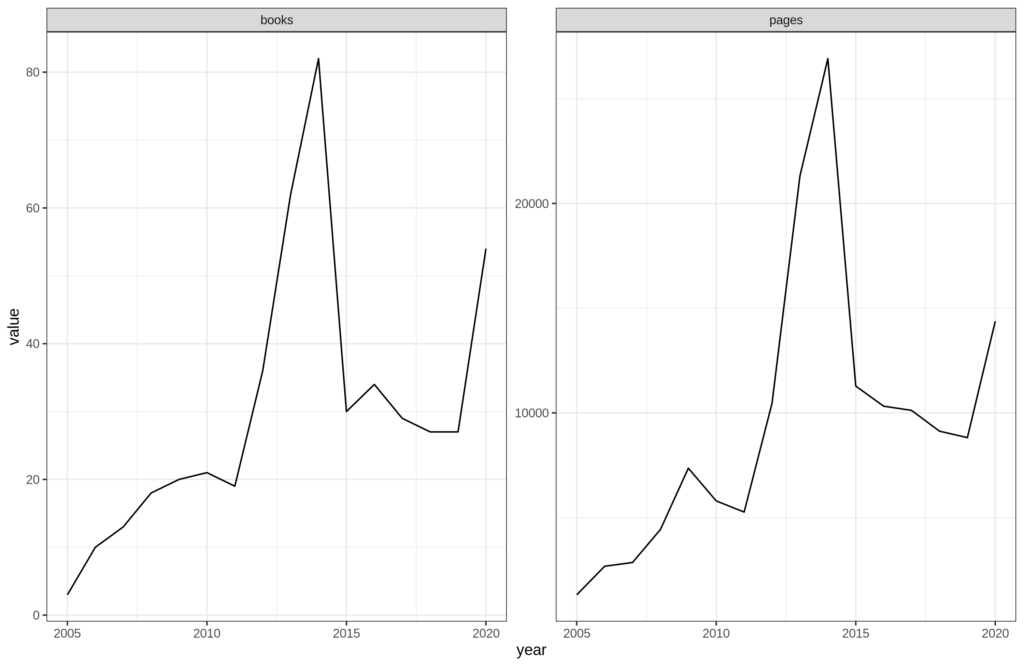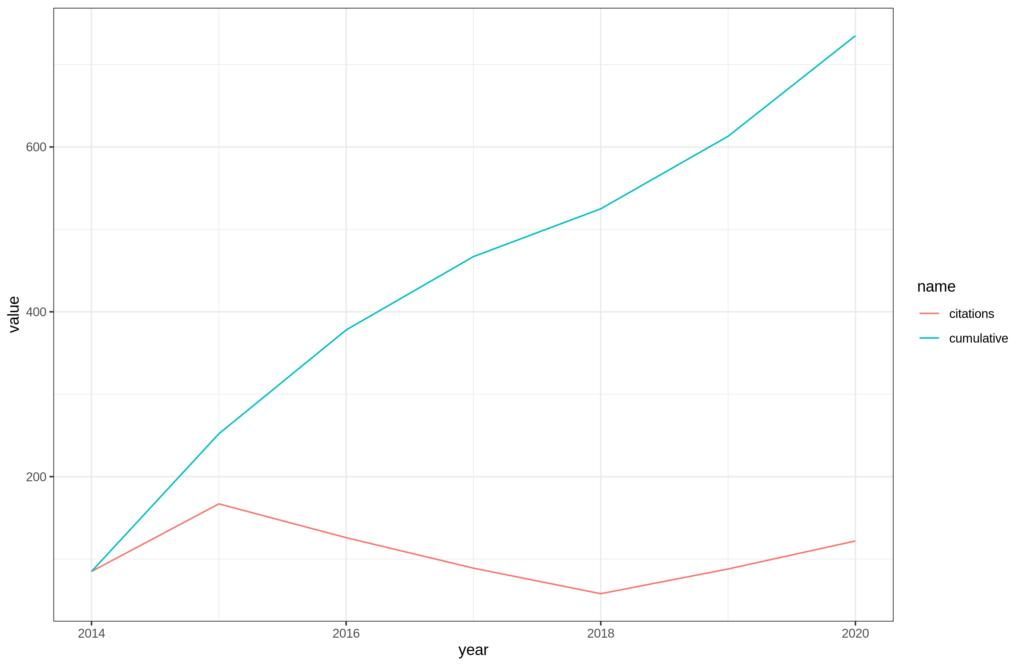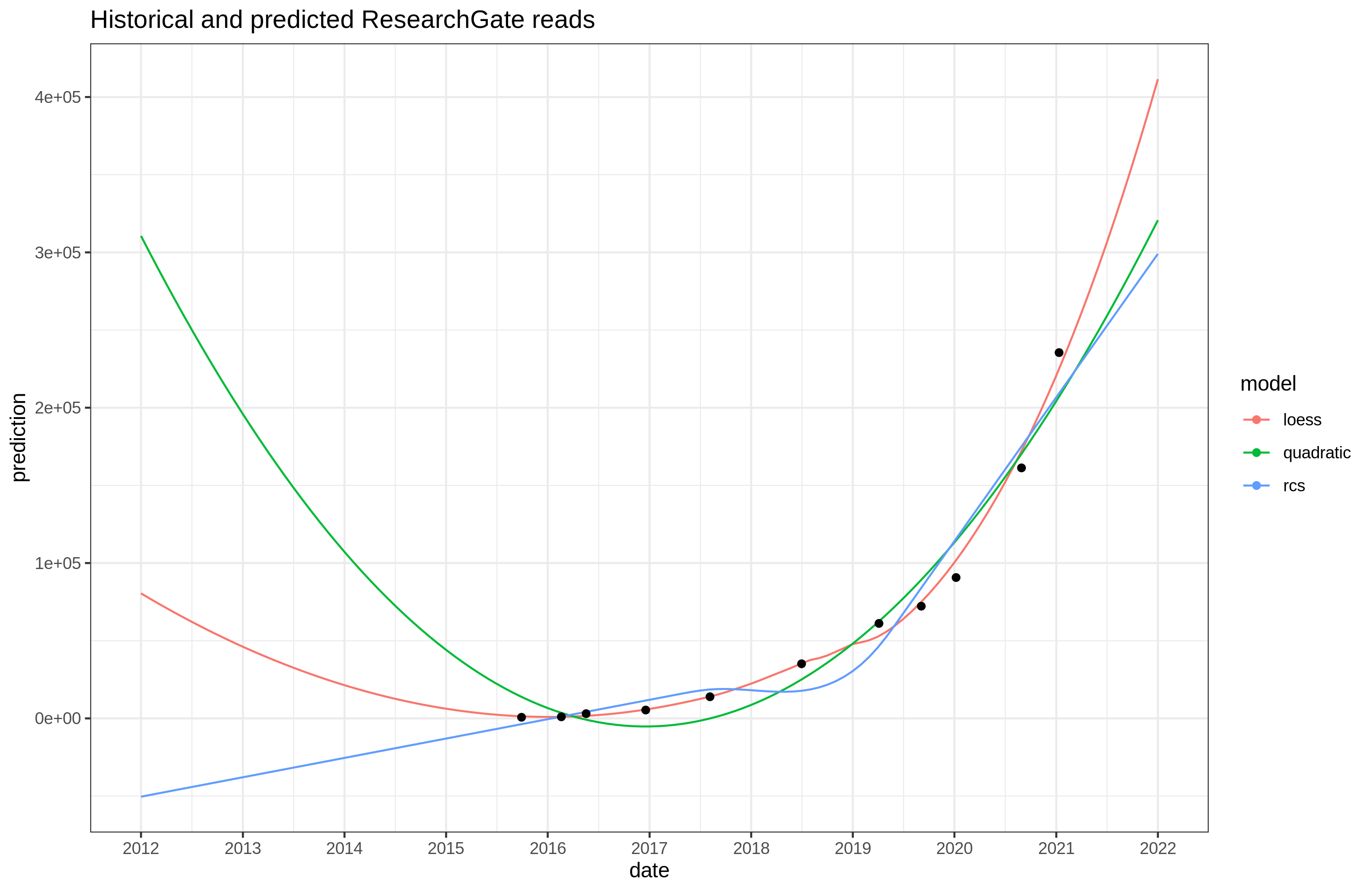## Goals and forecasts for 2021

These are goals and projections for my various productivity measures. These are all based on ignoring the tail risk of getting banned (which is now substantial across platforms).

The method of doing projections is not difficult: gather some prior data, eyeball the data, project with simple models, apply some adjustment for expected deviations (expect good/bad year to come).

### ResearchGate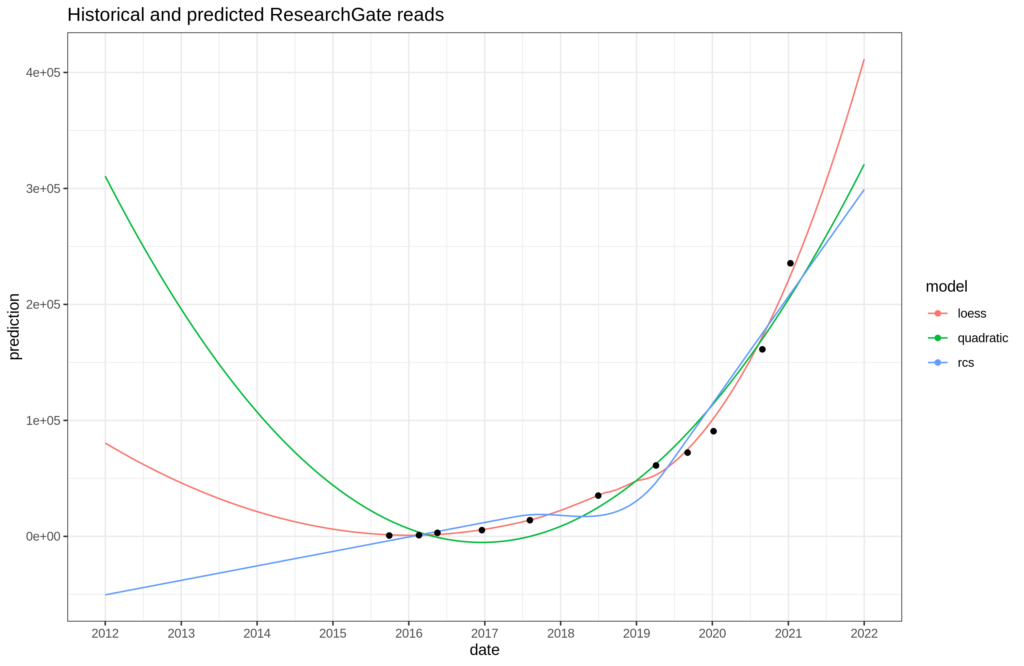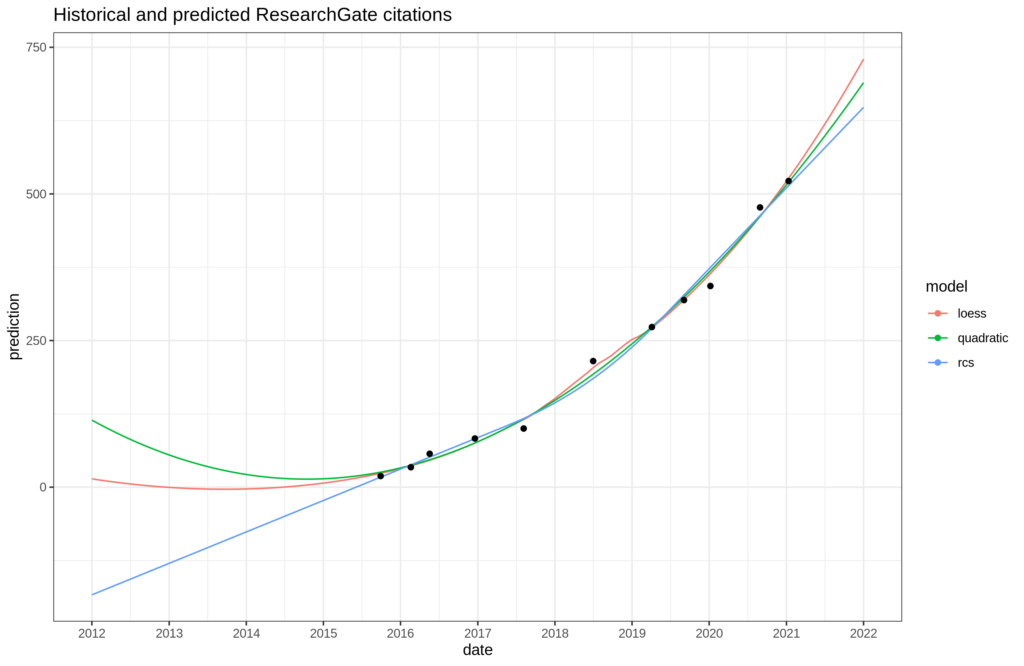I could have added an exponential model as well, but probably not much different from the quadratic one. In both cases, I predict values in between what the models suggest. This is essentially being function form agnostic, with roughly equal weight assigned to these models (Similar to Bayesian model averaging). I put the estimate at 349k [327k, 379k], and at 705 [639, 778]. The values in brackets are the 25% and 75% centiles of the probability distribution

(And no, we don’t care that the models produce nonsense results (below 0) in the past. We only care about the future.)The loess is clearly inappropriate. Otherwise, seems pretty linear, so I project 2.2k [1.9k, 2.6k].

### BlogpostsIn this case, the linear model is p > .05. This suggests that the best guess is just using the historical average, or the last value (if they are autocorrelated). I put the estimate at 85 [57, 113].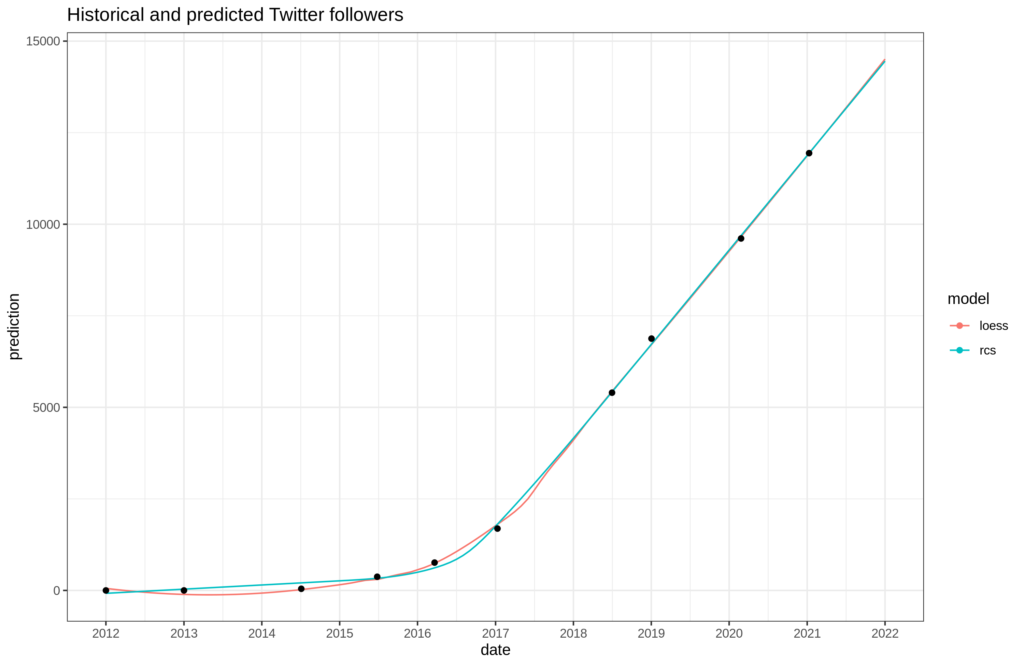The models are essentially equivalent. So we add some uncertainty, and I estimate 14.5k [14k, 15k].